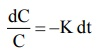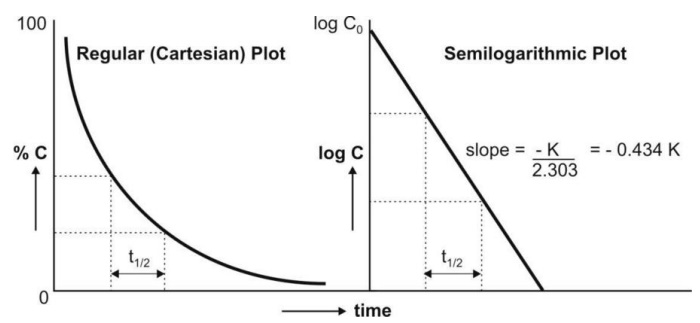# First-Order Kinetics (Linear Kinetics)

| Home | | Biopharmaceutics and Pharmacokinetics |

## Chapter: Biopharmaceutics and Pharmacokinetics : Pharmacokinetics Basic Considerations

first-order process is the one whose rate is directly proportional to the concentration of drug undergoing reaction i.e. greater the concentration, faster the reaction.

First-Order Kinetics (Linear Kinetics)

If n = 1, equation 8.4 becomes:

dC/dt = -KC            (8.10)

where K = first-order rate constant (in time–1 or per hour)

From equation 8.10, it is clear that a first-order process is the one whose rate is directly proportional to the concentration of drug undergoing reaction i.e. greater the concentration, faster the reaction. It is because of such proportionality between rate of reaction and the concentration of drug that a first-order process is said to follow linear kinetics (Fig. 8.3.).Fig. 8.3. Graph of first-order kinetics showing linear relationship between rate of reaction and concentration of drug (equation 8.10).

Rearrangement of equation 8.10 yields:

dC/C = -Kdt            (8.11)Integration of equation 8.11 gives:

ln C = ln C0 – Kt          (8.12)

Equation 8.12 can also be written in exponential form as:

C = C0 e- Kt              (8.13)

where e = natural (Naperian) log base.

Since equation 8.13 has only one exponent, the first-order process is also called as monoexponential rate process. Thus, a first-order process is characterized by logarithmic or exponential kinetics i.e. a constant fraction of drug undergoes reaction per unit time.

Since ln = 2.303 log, equation 8.12 can be written as:

log C = log C0 -  Kt/2.303          (8.14)

Or

log C = log C0 - 0.434 Kt          (8.15)

A semilogarithmic plot of equation 8.14 yields a straight line with slope = –K/2.303 and y-intercept = log Co (Fig. 8.4.).Fig. 8.4. Regular and semilog graphs of first-order kinetics